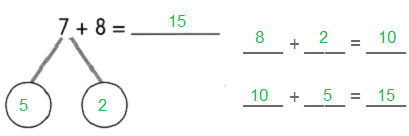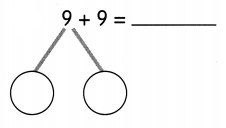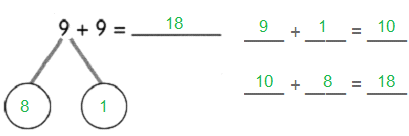Practice the problems of Math in Focus Grade 1 Workbook Answer Key Chapter 8 Practice 1 Ways to Add to score better marks in the exam.

Make a 10.

Example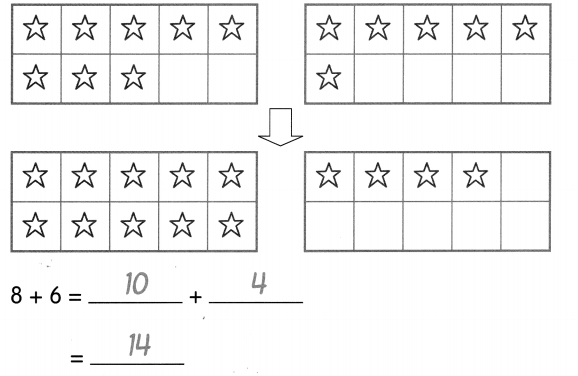Question 1.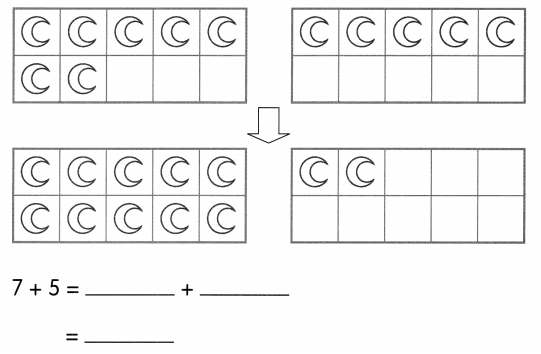7 + 5 = 10 + 2 = 12

Question 2.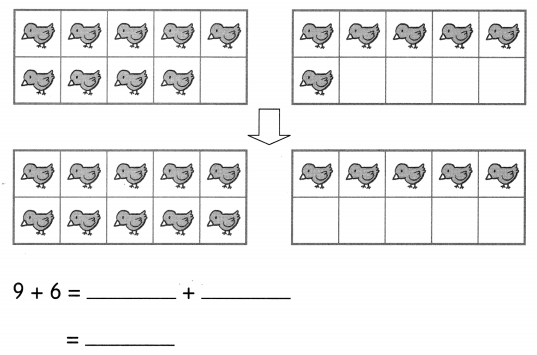9 + 6 = 10 + 5 = 15

Draw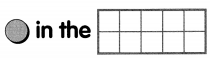to make a 10. Then add.

Example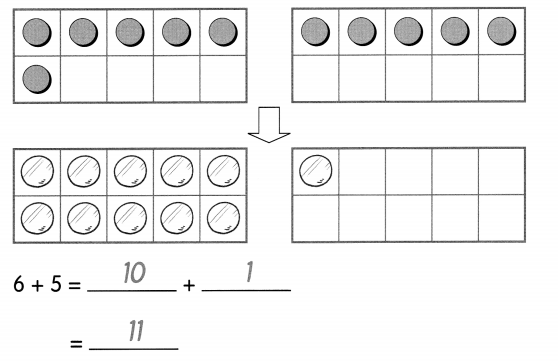Question 3.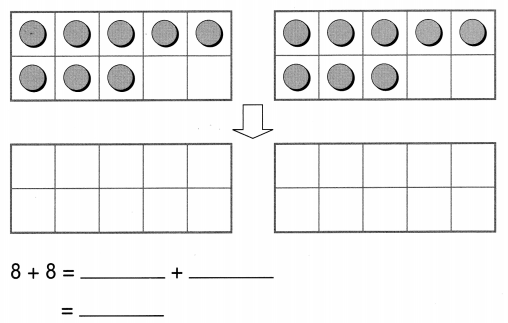Answer: 8 + 8 = 10 + 6 = 16

Question 4.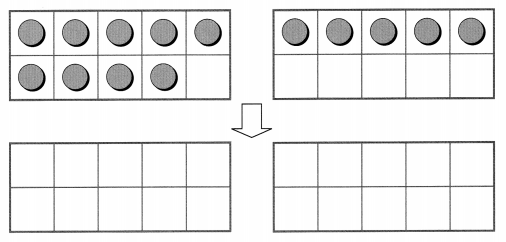9 + 5 = ___ + ___
= _____
Answer: 9 + 5 = 10 + 4 = 14

Question 5.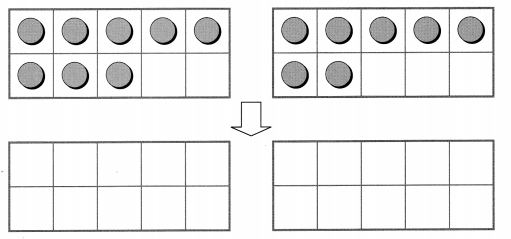8 + 7 = ___
= _____
Answer: 8 + 7 = 10 + 5 = 15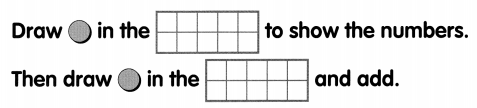Example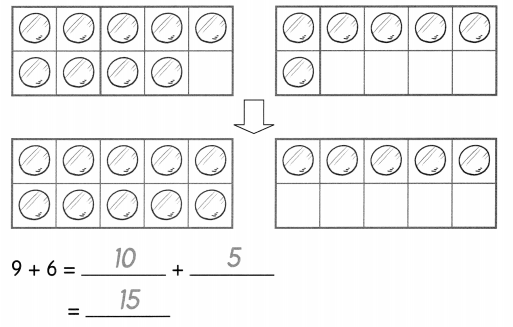Question 6.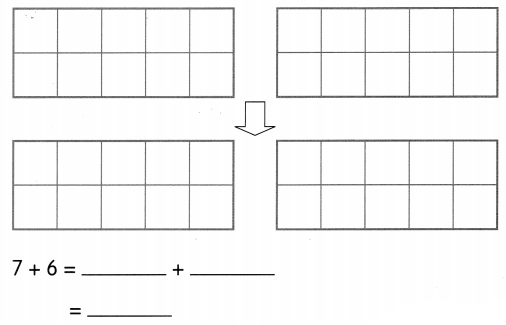Answer: 7 + 6 = 10 + 3 = 13

Make a 10.

Example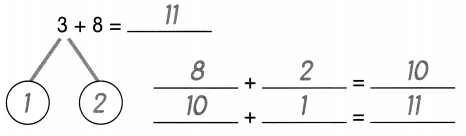Question 7.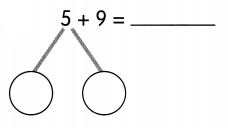____ + ____ = ____
____ + ____ = ____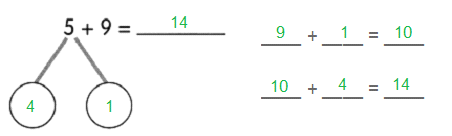Question 8.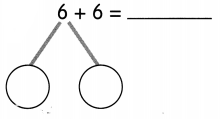____ + ____ = ____
____ + ____ = ____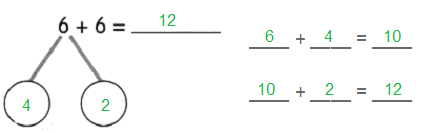Question 9.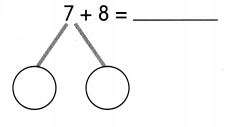____ + ____ = ____
____ + ____ = ____